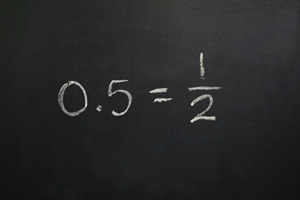On teaching fractions with a number line
THURSDAY, MAY 28, 2015 11:35 AMThe Common Core State Standards mentions the use of a number line in many of its mathematical Standards, but it's especially prevalent in the parts of the CCSS that deal with fractions. Beginning in third grade, the Common Core asks students to represent fractions on a number line, and in later grades, the tool can be used to better understand how to add, subtract, multiply and divide partial numbers. But why use a number line?

Beginning in kindergarten and first grade, number lines are an effective way for students to visualize numbers and their relationships. For instance, in a simple addition problem like 9 + 5, a number line can easily show that 9 equal segments plus 5 equal segments add up to a total of 14 sections of the line. This visualization is very important for learning about fractions. Here's how:

Teaching students what fractions are
Children are taught early on that numbers are representative of whole objects, like cookies on a plate or apples in a store. But partial numbers are a much harder concept to teach using objects. So, consider a number line that consists of 5 segments between 0 and 5. Students can clearly see that there is a whole number between 2 and 4. But a number line also shows something else: There are also numbers between, say 2 and 3 or 3 and 4. This visual representation of fractions is easy for students to understand.

Teaching students to operate using fractions
Because number lines show fractions in a visual way, they also help students learn how to perform basic operations with fractions, which is a commonly difficult concept for children to grasp. Think about how complicated this is for students: 2 + 2 is equal to 4, right? So why would 1/2 + 1/2 not equal 1/4? On a number line, though, two halves of a segment clearly add up to a whole one, so 1/2 + 1/2 must equal 2/2 or 1.

Even if you make things a little more complicated than basic addition, using number lines to understand fractions still holds up. For instance, to divide a number by a fraction, you must change the fraction to its reciprocal and multiply. But part of teaching Common Core math is helping students learn why they're doing certain operations, and the number line can provide this answer. Try dividing 10 by 1/2. Though students may think of the equation as dividing 10 in half for an answer of 5, the correct response is actually 20. Do the problem on a number line: Divide 10 segments into halves. Now count the halves, and you have 20 new segments. So, the clear solution is 20. You also get this answer when multiplying 10 by 2/1 (the reciprocal of 1/2).RELATED ARTICLES All About TerraNova 3 Testing Teaching gifted kids in normal classes Helping your kids continue learning this summer More..
 NEWS CATEGORIES NEWS ARCHIVE